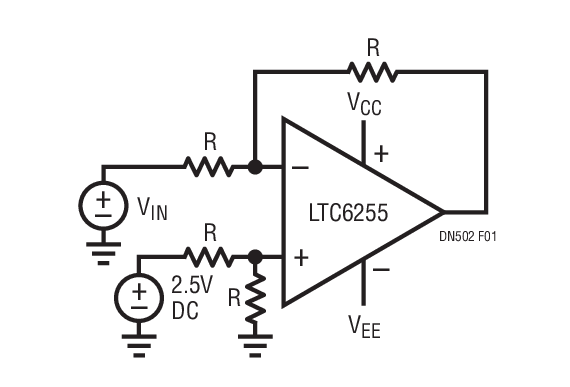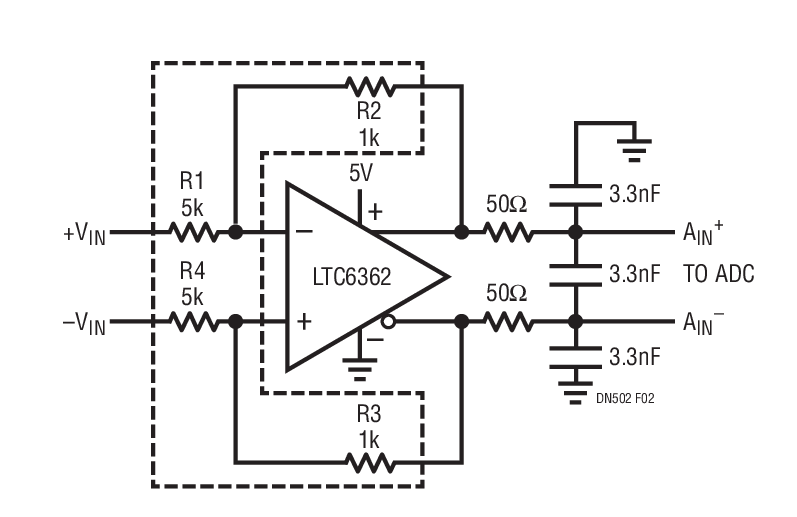# Matched Resistor Networks for Precision Amplifier Applications

### Introduction

Some ideal op amp configurations assume that the feedback resistors exhibit perfect matching. In practice, resistor non-idealities can affect various circuit parameters such as common mode rejection ratio (CMRR), harmonic distortion and stability. For instance, as shown in Figure 1, a single-ended amplifier configured to level-shift a ground-referenced signal to a common mode of 2.5V needs a good CMRR. Assuming 34dB CMRR and no input signal, this 2.5V level shifter exhibits an output offset of 50mV, which can even overwhelm the LSB and offset errors of 12-bit ADCs and drivers.Figure 1. A Single-Ended Op Amp Used as a Level Shifter

For an op amp, 34dB is a less than ideal CMRR. However, a feedback network of 1% tolerance resistors can limit the CMRR to 34dB regardless of the op amp’s capabilities. Highly matched resistors, such as those provided by the LT5400, available in 0.01%, 0.025% and 0.05% matching, ensure that the designer can approach or meet amplifier data sheet specifications. This design note compares the LT5400 with thick film, 0402, 1% tolerance surface mount resistors. CMRR, harmonic distortion and stability are considered with these resistors for feedback around an LTC6362 op amp, as shown in Figure 2.Figure 2. Fully Differential Op Amp Configured for VOUT/VIN = 0.2

### Common Mode Rejection Ratio

In order to obtain precise measurements in the presence of common mode noise, a high CMRR is important. Input CMRR is defined as the ratio of differential gain (VOUT(DIFF)/VIN(DIFF)) to the input common mode to differential conversion (VOUT(DIFF)/VIN(CM)).

In ideal single-ended and fully differential amplifiers, only the input differential level affects the output voltage. However, in real circuits, resistor mismatch limits the available CMRR. Consider this circuit configured to attenuate a ±10V signal to a ±2V signal. Using typical surface mount resistors with 2% matching (1% tolerance), the worst-case CMRR contribution from the resistors is 30dB. With 0.01% tolerance, 0.02% matching, the worst-case CMRR contribution from the resistors is 70dB. A limiting factor in the CMRR equation is:

This expression reduces to the resistor matching ratio for typical resistors, but the LT5400 takes an additional step to offer improved CMRR by constraining the matching between resistor pairs R1/R2 and R4/R3. By defining this equation as matching for CMRR, the LT5400 offers accuracy that’s better than just the resistor matching ratio. For example, the LT5400A guarantees:

which improves worst-case CMRR to 82dB.

A bench test of the circuit yielded a CMRR of 50.7dB (highly resistor matching limited) with 1% tolerance resistors, and 86.6dB with the LT5400. In this case, a 2.5V common mode input would result in an offset of 1.5mV with 1% thick-film resistors and an offset of 23μV with the LT5400, making it suitable for 18-bit ADC applications where DC accuracy is critical.

### Harmonic Distortion

Harmonic distortion is also important when choosing resistors for a precision application. A large-signal voltage across a resistor may significantly change the resistance depending on the size and material. This problem occurs in a number of chip-based resistors, and naturally becomes more severe as the power levels at the resistors increase. Table 1 compares the distortion of thick film, through-hole, and the LT5400 resistors based on high power drive and similar power drive. The results suggest that for a given signal, the LT5400 distorts the signal much less than other resistor types.

### Stability

Figure 3 shows a model of the distributed capacitance between resistors in the LT5400. To achieve high precision matching and tracking in the LT5400, many small SiCr resistors are confi gured in series and parallel. As a result of the complex interdigitation, the LT5400 resistors can be modeled as a series of infinitesimal resistors with parasitic capacitance between adjacent segments and between individual segments and the exposed pad. In contrast, typical surface mount resistors, without the tight layout, typically exhibit significantly less parasitic capacitance.Figure 3. A Simple Model of the Distributed Capacitance in a Matched Resistor IC. The Sum of R′ Components Creates an Equivalent Single Resistor. The Net Effect of C′INTER is 1.4pF and the Net Effect of C’EXPOSED is 5.5pF

The effect of inter-resistor capacitance can be mitigated when the exposed pad is grounded. However, even after grounding the exposed pad, this capacitance still affects circuit stability by forming a parasitic pole on the order of the total resistance times the total capacitance.

Since overshoot is inversely proportional to phase margin, minimizing step response overshoot is a good way to ensure circuit stability. The uncompensated LT5400 configuration exhibits 27% compared to 17% overshoot from the 0402 configuration. However, the compensation capacitor necessary to achieve 8% overshoot is approximately the same in both confi gurations: 18pF with the LT5400; 15pF with 0402 resistors. With nearly identical compensation, the two circuits display similar stability characteristics.

### Conclusion

The actual performance of precision amplifiers and ADCs is often difficult to achieve since data sheet specifications assume ideal components. Carefully matched resistor networks, such as those supplied by the LT5400, enable precision matching orders of magnitude better than discrete components, ensuring data sheet specifications are met for precision ICs.